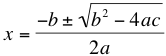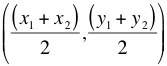# Math Help: Formulas to Memorize

09/21/2015

## Math Formulas You Should Know

Because math builds upon what you already know, it helps to memorize formulas for problems that come up often. Here are some math formulas you will want to commit to memory.

### Rectangle Formulas

Area=lw l is the length and w is the width. Multiply them together to find the area of a rectangle. Perimeter=2l+2w Double the length of each side and add the results together to calculate the perimeter. Volume=lwh h is the height of the rectangle. Multiply length, width, and height to find the volume.

### Parallelogram

To find the area of a parallelogram, drop two right triangles to determine the heights and turn the parallelogram into a rectangle.

### Quadratic FormulaSolve any quadratic equation in the form ax^2+bx+c=0 with this formula. For those formulas that are difficult to find the difference of squares, or if you are in a rush on an exam, the quadratic formula will be very nice to know.

### Midpoint FormulaWhen you need to find the midpoint of a line, use this formula to determine the coordinates.

### Slope FormulaTo determine the slope of a line, choose two points on the line and divide the rise (how much the line goes up or down) by the run (how much the line goes left to right).

### Statistical Combinations

To find the total possible different combinations of different elements, simply multiply them all together. For example, if there are 4 appetizers, 5 entrees, and 3 desserts on a menu, there are 4*5*3=60 different possible combinations.

## For Tutoring in Southgate

If you would like additional help with math, contact The Tutoring Center in Southgate. We have one-to-one tutoring programs in math, reading, writing, and test prep. Visit us online to learn more and call 734-785-8430 to schedule a free diagnostic assessment.

### FREE CONSULTATION

Schedule your Free Diagnostic Assessment Today!
Learn more about
THE TUTORING CENTER
on the national website: